Tate conjectures

Conjecture 1. If the fieldis finitely generated over its prime subfield, ifis a smooth projective variety over, ifis a prime number different from the characteristic of the field, if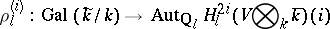is the natural-adic representation, and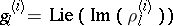, then the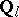-space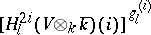, the space of elements of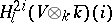annihilated by, is generated by the homology classes of algebraic cycles of codimensionon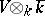(cf. also Algebraic cycle).
Conjecture 2. The rank of the group of classes of algebraic cycles of codimensiononmodulo homology equivalence coincides with the order of the pole of the functionat the point.
These conjectures were verified for a large number of particular cases; restrictions are imposed both on the fieldand on the variety.